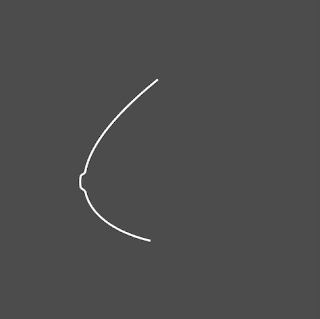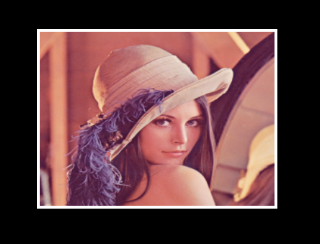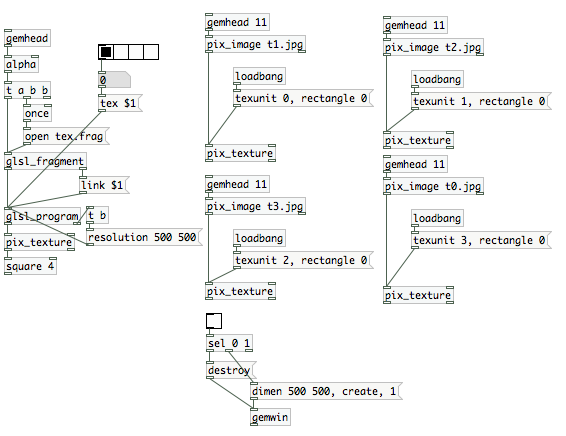## 2013年12月9日 星期一

### [教學]以數學建構人體曲線2013/12/13 補充

• x=0 to 1
• y=0 to 1
• z=11*x*log(x)*y*(y-1)+exp(-((25*x-25/e)^2+(25*y-25/2)^2)^3)/25

3d版本 Download，可能需要的擴充物件

## 2013年11月24日 星期日

### [教學] 網站範例檔當中，遺失的[k_xxxx ] 物件（圖一）

（圖二）

(補充：20140822)

(補充：20170717)
dropbox 空間已死，所以k_obj全都移到github上了，請到這裡下載使用
https://github.com/aluanwang/k_obj

### [音像實驗室] 2/30 他將點醒我

In this series of "Audiovisual Lab", unlike previous style of thinking along the way of doing, all works in this series would begin with a draft, then implement the draft accordingly. By doing so, first of all, I can examine my competency and understand the insufficient of my ability. Secondly, by planning in advance, to avoid similarity among 30 experiment.

In this experiment, many square zones shall be drawn to form different triggering zones. When the time axis meets the zone, it makes sounds. The interval axis will keep moving back and forth. The final result is like a sequencer drum machine.

## 2013年11月17日 星期日

### [音像實驗室] 1/30 電流急急棒

In this experiment, the red/green line pulled out at the beginning decides the pitch of the sound (the longer the line, the higher the pitch). The following distance between the control point and the red/green line decides the amplitude of the sound (the farther the distance, the louder the sound). Finally, the prportion between the distance between the crossover point reflected by the control point to the red/green line and the ends of the red/green line decides the length of the sound.

## 2013年11月3日 星期日

### 「Pd筆記-68」 清除win安裝的預設值## 2013年10月25日 星期五

### [GLSL]入門-5 用binary（2進制）來繪製點陣圖形

shader是無法載入字體的，但假使有一天我們想要在shader裡面秀出文字，這時候便可以透過，把文字轉成2 維矩陣方塊的方式（點陣字體），載入進shader。

```uniform vec2 iResolution;
uniform float pattern;

float bit(float n, vec2 p) {
p.x=(floor(p.x*5.0));
p.y=(floor(p.y*5.0));
float k = (p.x + (5.0*p.y));
if (int(mod(n/(exp2(k)), 2.0)) == 1){
return 1.0;
} else{
return 0.0;
}

}
void main()
{
vec2 uv = gl_FragCoord.xy/iResolution.xy;
uv.y=1.0-uv.y;

vec2 uv2=mod(uv*5.0,1.0);
vec3 col2;
if((uv2.x<0 .95="" uv2.x="">0.05)  && (uv2.y<0 .95="" uv2.y="">0.05) ){
col2=vec3(1.0,1.0,1.0);
}else{
col2=vec3(0.3,0.3,0.3);
}
vec3 col = vec3(bit(pattern, uv)*floor(mod(gl_FragCoord.x/2.0,2.0)) * floor(mod(gl_FragCoord.y/2.0,2.0)));
gl_FragColor = vec4(col*col2,1.0);
}
```
shader code

Download

## 2013年10月17日 星期四

### [表演]失聲祭76

http://lsf-taiwan.blogspot.tw/2013/10/lsf76.html

2013, October, 19 (Sat) ＠噪咖

「跟混種的演出稍稍的不同，加強了GUI的設計，以及繪圖操控。」

[Squaring the circle]

## 2013年9月23日 星期一

### [混種現場]音像之夜

http://registrano.com/events/91d176

http://registrano.com/events/d8fa94

## 2013年9月9日 星期一

### [演出]混種現場X睡不著-音像系列

fb活動頁：https://www.facebook.com/events/384685454993429/

## 2013年8月28日 星期三

### [GLSL] 入門-4 翻轉影像```uniform sampler2D tex;
uniform vec2 flip;

void main(void){

vec3 color=texture2D(tex,abs(flip-gl_TexCoord.xy)).rgb;
//透過外部輸入的2維flip變數，重新排列影像pixel的順序。

color += gl_TexCoord.x>0.99 ? 1.0:0.0;//右邊界畫白線
color += gl_TexCoord.x<0.01 ? 1.0:0.0;//左邊界畫白線
color += gl_TexCoord.y>0.985 ? 1.0:0.0;//上邊界畫白線
color += gl_TexCoord.y<0.015 ? 1.0:0.0;//下邊界畫白線

gl_FragColor=vec4(color,1.0);
}
```

download

## 2013年8月26日 星期一

### [GLSL] 入門-3 把貼圖的材質當成陣列使用

```uniform sampler2D tex;
uniform vec2 resolution;
uniform float chsize;

void main(void){
vec2 uv = gl_FragCoord.xy / resolution.xy;
vec2 center = gl_FragCoord.xy / resolution.xy * 2.0-1.0;

float k = (gl_FragCoord.x+(gl_FragCoord.y*800.0))/(800.0*600.0);
vec3 col=texture2D(tex,vec2(mod(mod(uv.x*chsize,1.0)*chsize,1.0) ,
mod(mod(uv.y*chsize,1.0)*chsize,1.0)
)).rgb;
float f=col.r;
gl_FragColor = vec4(f,f,f,1.0);
}

```

## 2013年8月23日 星期五

### [GLSL] 入門-2 透過glsl載入buffer影像

```uniform sampler2D tex;
uniform vec2 resolution;
uniform int f;

void main (void)
{

vec2 uv=gl_FragCoord.xy/resolution.xy;
vec3 col= texture2D(tex, vec2(uv.x , 1.0-uv.y)).xyz;

gl_FragColor = vec4( col, 1.0 );

}
```file download

## 2013年8月6日 星期二

### [GLSL] 入門-1 矩陣圓球### [example 1] ↓

```//沒有光暈的球
uniform vec2 resolution;
uniform float time;
vec2 uv;

vec2 circle(float i){
return vec2(uv+vec2(fract(i/10.0)*2.0-0.9 ,
fract(floor(i/10.0)/10.0)*2.0-0.9 ));
}

void main(void){
uv = (gl_FragCoord.xy / resolution.xy)*2.0-1.0;
float col;
float k;

for(float i=0.0;i<100 .0="" 1.0:0.0="" col="" gl_fragcolor="vec4(col,col,col,1.0);" i="" k="" pre="">
```### [example 2]

```//有光暈的球
uniform vec2 resolution;
uniform float time;
vec2 uv;

vec2 circle(float i){
return uv-vec2(fract(i/10.0)*2.0-0.9 ,fract(floor(i/10.0)/10.0)*2.0-0.9 );
}

void main(void){
uv = (gl_FragCoord.xy / resolution.xy)*2.0-1.0;
float col;
float back=abs(time-0.5);

for(float i=0.0;i<100 .0="" back="" circle="" col="" gl_fragcolor="vec4(col,col,col,1.0);" i="" length="" pow="" pre="">
```
example download

### [工作坊] Pd-VJing 第七週

VJ實體控制器中，常常可以見到機器中間會出現一個橫軸的滑桿，我們稱呼為cross fader，它通常是用來切換兩個不同的影像素材。本範例則將透過自製的PD界面來模擬此效果。

Example Download

Example Download

## 2013年7月26日 星期五

### 「Pd筆記-64」 gridflow安裝修正

http://gridflow.ca/

/Library/pd

## 2013年7月6日 星期六

### [表演]Good Vibration，好抖～

6/30以前購票還有早抖優惠，位置有限，先抖先贏！

## 2013年6月23日 星期日

### 「筆記」ＱＣ進階筆記 4-1

```var clicked=false;
var control={};
var clock=[];

function dis(x1,y1,x2,y2){
return Math.sqrt(Math.pow((x1-x2),2)+Math.pow((y1-y2),2));
}

function angle(x,y,x1,y1){
return Math.atan2(y1-y,x1-x);
}

function (__structure outlet,__structure outlet2) main (__number x,__number y,
__boolean click,__boolean reset,__number time)
{

for(var i=0;i<clock.length;i++){
clock[i].theta+=clock[i].r/10;
clock[i].x1=clock[i].x+Math.cos(clock[i].theta)*clock[i].r;
clock[i].y1=clock[i].y+Math.sin(clock[i].theta)*clock[i].r;
}

var result = new Object();
//if(click==true && clicked==false)
if(reset){
clock=[];
}

if(click && !clicked){
clicked=true;
control.posx=x;
control.posy=y;
control.enable=true;
}
if(click && clicked){
control.posx1=x;
control.posy1=y;
control.dis=dis(control.posx,control.posy,control.posx1,control.posy1);
control.angle=angle(control.posx,control.posy,control.posx1,control.posy1)
}
if(!click && clicked){
clicked=false;
control.enable=false;

clock.push({
x:control.posx,
y:control.posy,
x1:control.posx1,
y1:control.posy1,
r:control.dis,
theta:control.angle,
});
}
result.outlet=control;
result.outlet2=clock;
return result;
}
```

(draw line)

（iterator)

qc2-w4-1.qtz